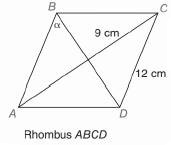Chapter 11.CR, Problem 7CR### Elementary Geometry for College St...

6th Edition
Daniel C. Alexander + 1 other
ISBN: 9781285195698

#### Solutions

Chapter
Section### Elementary Geometry for College St...

6th Edition
Daniel C. Alexander + 1 other
ISBN: 9781285195698
Textbook Problem
24 views

# In Exercises 5 to 8, state the ratio needed, and use it to find the measure of the indicated angle to the nearest degree.To determine

To find:

The measure of the angle indicated to the nearest degree.

Explanation

Procedure used:

In any right triangle ABC with mA=θ we have the following ratios.

sinθ=OppositeHypotenuse

Calculation:

Given:

The above given figure is a rhombus.

By the properties of a rhombus, all of its sides are equal in length, the diagonals intersect each other at right angle and each of the diagonal bisect the angle at the vertices, we have

AB=BC=CD=DA=12 cm,

mAOB=BOC=COD=DOA=90°, and

mABO=mCBO=α

Here, ‘O’ is the point of intersection of the diagonals AC and BD

### Still sussing out bartleby?

Check out a sample textbook solution.

See a sample solution

#### The Solution to Your Study Problems

Bartleby provides explanations to thousands of textbook problems written by our experts, many with advanced degrees!

Get Started

#### Find more solutions based on key concepts